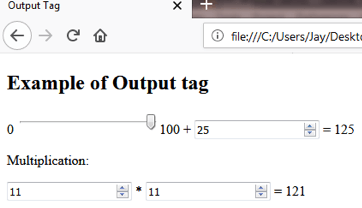Output Tag in HTML || HTML Output Tag# HTML OUTPUT <output> Tag

2143

HTML Output <output> tag is for showing the result of a calculation performed by a script or it could show the output of a user's interaction by using <form> element.

The <output> tag is always used in pairs. It should always have the closing tag and starting tag.

It is a new tag in HTML5.

Program:

```<!DOCTYPE html>
<html>
<title> Output Tag </title>
<body>
<h2>Example of Output tag</h2>
<form oninput="x.value=parseInt(a.value)+parseInt(b.value)">0
<input type="range" id="a" value="50">100 +
<input type="number" id="b" value="25"> =
<output name="x" for="a b"></output>
</form>
<p>Multiplication:</p>
<form oninput = "d.value = e.valueAsNumber * f.valueAsNumber">
<input type = "number" id = "e"> *
<input type = "number" id = "f"> =
<output name = "d" for = "e f"> </output>
</form>
</body>
</html>```

Output:Share:## Big Hosting Days

Upto 75@ OFF on Web Hosting

## Get a .COM for just \$6.98

Secure Domain for a Mini Price